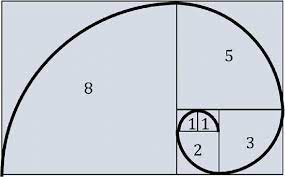## Fibonacci & the Golden Ratio

I guess you must be wondering what Fibonacci is. Actually, it’s not a what, it’s a who.

Fibonacci was an Italian mathematician; one of the smartest mathematicians of all time.

Have you ever wondered if there is a universal code to the entire universe? That we are all just a part of an algorithm, bits and bytes conformed together to make somewhat meaningful matter?

1,1,2,3,5,8,13,21. What you are seeing here are the first few numbers of the Fibonacci sequence. A recursion relation that amazed me to my core. Any following number comes from the addition of the two previous numbers. Once you have taken time to confirm that, get ready, for an intellectual journey like no other.

The Fibonacci Spiral is a representation of the sequence itself. Imagine drawing squares that are in scale with the numbers. One small square and then adding more, adjacent to each other.  You will end up with a rectangle. By drawing a line, starting from the first Fibonacci number and arcing across the squares, you end up with this spiral. You may think that this is just an ordinary spiral, that I am another crazy mathematician making up a story to fascinate the masses. Hold on, the best is yet to come.The Golden Ratio, also known to some as the Divine Proportion can be explained using a line segment. We divide a line segment into two parts and assume that one of the segments (x) is larger than the other (y). We also assume that the larger segment over the smaller (x/y) is the same as the total length of the segment (x+y) divided by the larger segment (x). Given these assumptions, the Golden Ratio are these ratios (x/y) and (x+y/x). The Golden Ratio is represented by the Greek letter Phi 𝚽. You can also understand this with the Golden Rectangle. It is a rectangle that has sides in the ratio of the Golden ratio. The longest side of the rectangle divided by the shortest side will be equal to the Golden Ratio. When the Golden Rectangle is constructed, you will see a set of spiralling squares like this.What you are seeing here is the Golden Spiral, a logarithmic spiral that fits perfectly within the Golden Rectangle. The radius of the Golden Spiral increases by a factor of the Golden Ratio each one-quarter of a turn. You must be thinking that the Fibonacci Spiral and the Golden Spiral are the same. However, even though they look very similar, they are not the same. The Fibonacci Spiral starts from the middle, spiralling outwards. The Golden Spiral on the other hand starts from the outside, spiralling in towards a point. However, as the Fibonacci Spiral moves outwards, the ratio of the adjacent Fibonacci numbers approaches the Golden Ratio. So in essence, the Fibonacci Spiral becomes the Golden Spiral. This spiral is not just some boring mathematical phenomenon that appears only on paper and does not exist outside its assumptions. It appears in nature, all around us, inevitably and indefinitely incorporated in ways you could not even imagine. The spiralling seeds on a sunflower head follow the Fibonacci Spiral! Snail shells and nautilus shells are wound in that way as well. Our inner cochlea in our ears follows the Fibonacci Spiral. Hurricanes, which you would assume were just a mass of chaos, twist in a Fibonacci Spiral. The next time you enjoy a pineapple, be sure to notice the Fibonacci Spiral on its skin!

You may be thinking that these are just ordinary spirals- that I am exaggerating their importance. However, it is not just the spirals that exist in nature; the Fibonacci sequence and the Golden Ratio itself appear in nature. The petals on many flowers are placed at angles of a fraction of 𝚽, which maximizes their exposure to sunlight! The way a tree’s branches usually split follows the Fibonacci sequence. The ratio of female to male honey bees in a colony always approximates to the Golden Ratio. The Fibonacci numbers can be found in the microscopic realm as well! The DNA molecule measures 34×21 angstroms long for each full cycle of its double helix, both of which are Fibonacci numbers and whose ratio approximates to the Golden Ratio. The galaxies themselves spiral in a Fibonacci spiral. This leaves us with nagging questions we cannot ignore. How could we possibly find so many appearances of this sequence in nature? Doesn’t a coincidence become reality when it happens frequently? Are there coincidences in our world, or is that just another excuse to ignore the extraordinary? Maybe we are all just pawns in a greater game, a game that we do not know we are playing. If there can be order to the chaos of a hurricane, then is there actual chaos or is there just orderly chaos we are too mundane to identify? These phenomena speak to a deeper underlying current in the universe. It shows us that no matter what we are, human or pineapple, we all have a connection, an invisible string threading through the very fabric of our existence. Maybe the Divine Proportion is really divine. Maybe these small recurring appearances are bread crumbs left for us, a divine puzzle we as humans, exist to solve. Our reference to God having a plan for all of us is a nod to the belief that we all follow a predestined path, guided unknowingly. For me, studying the Fibonacci Numbers and the Golden Ratio showed me that I always need to look at the bigger picture. To be mindful of my surroundings; to look beyond the surface of a person or a problem in order to truly understand its meaning. The next time you see a sunflower, I hope you see past its beauty to the mathematical wonder beyond.